Скачать презентацию Day 6 What we will do today

e61668b313fe35c4e84a028b2651d7e0.ppt

• Количество слайдов: 55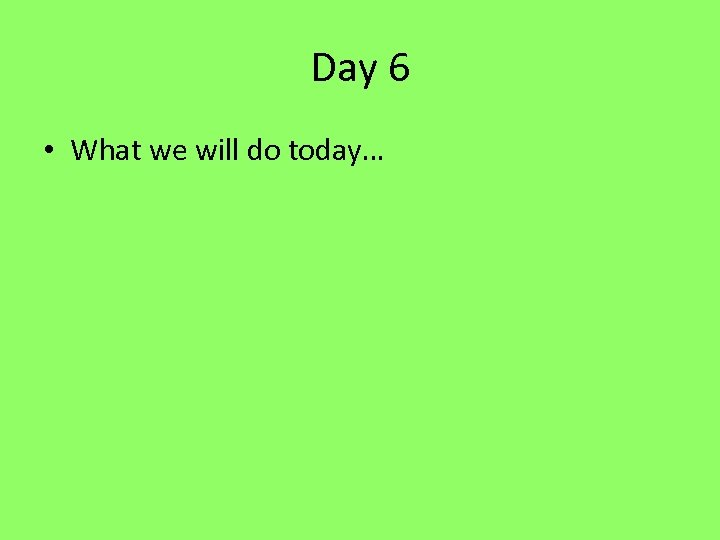Day 6 • What we will do today…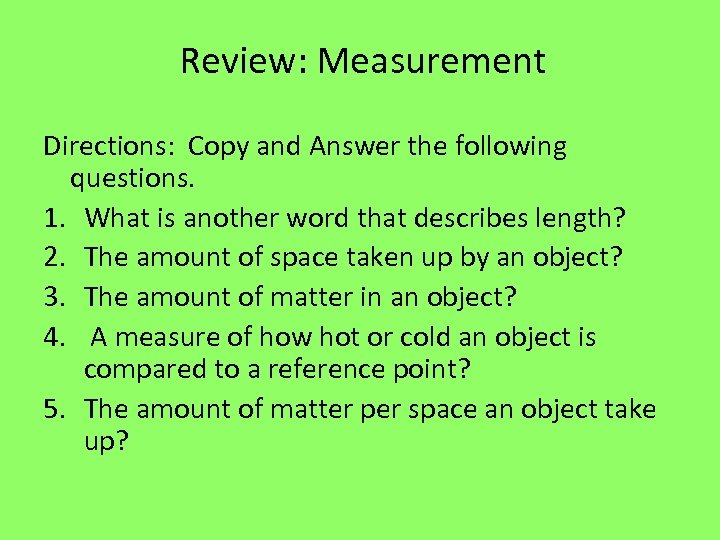Review: Measurement Directions: Copy and Answer the following questions. 1. What is another word that describes length? 2. The amount of space taken up by an object? 3. The amount of matter in an object? 4. A measure of how hot or cold an object is compared to a reference point? 5. The amount of matter per space an object take up?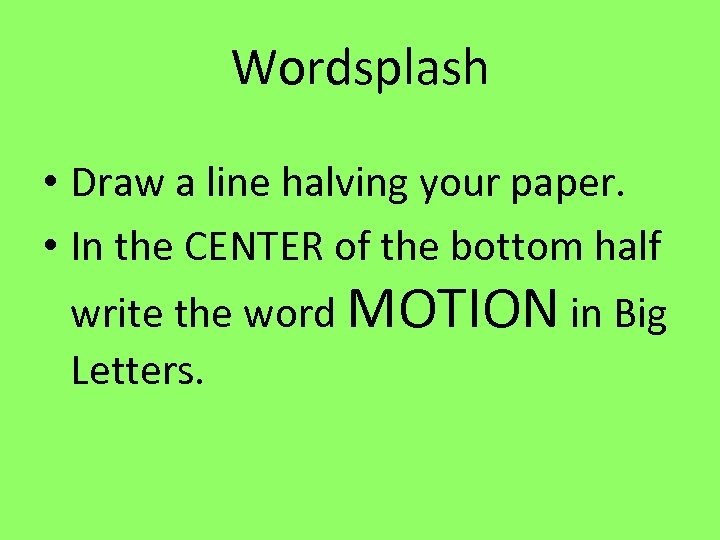Wordsplash • Draw a line halving your paper. • In the CENTER of the bottom half write the word MOTION in Big Letters.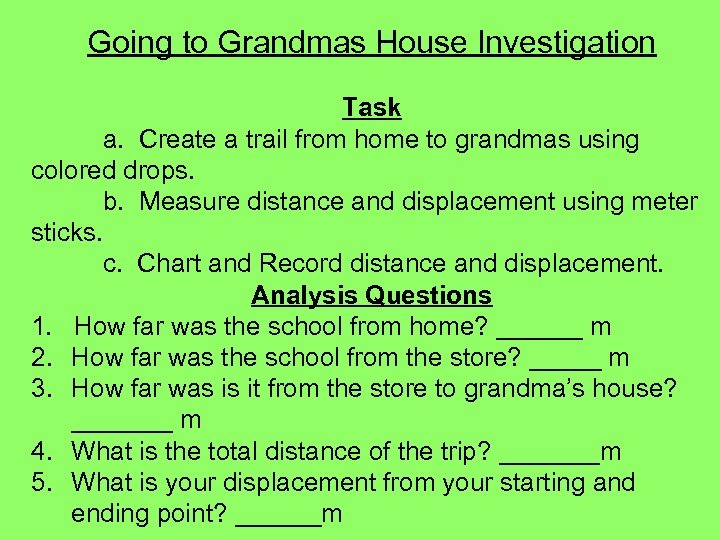Going to Grandmas House Investigation Task a. Create a trail from home to grandmas using colored drops. b. Measure distance and displacement using meter sticks. c. Chart and Record distance and displacement. Analysis Questions 1. How far was the school from home? ______ m 2. How far was the school from the store? _____ m 3. How far was is it from the store to grandma’s house? _______ m 4. What is the total distance of the trip? _______m 5. What is your displacement from your starting and ending point? ______m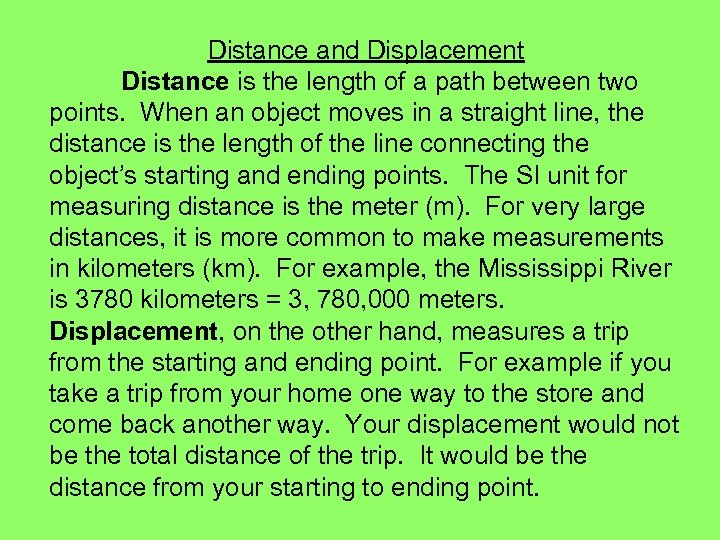Distance and Displacement Distance is the length of a path between two points. When an object moves in a straight line, the distance is the length of the line connecting the object’s starting and ending points. The SI unit for measuring distance is the meter (m). For very large distances, it is more common to make measurements in kilometers (km). For example, the Mississippi River is 3780 kilometers = 3, 780, 000 meters. Displacement, on the other hand, measures a trip from the starting and ending point. For example if you take a trip from your home one way to the store and come back another way. Your displacement would not be the total distance of the trip. It would be the distance from your starting to ending point.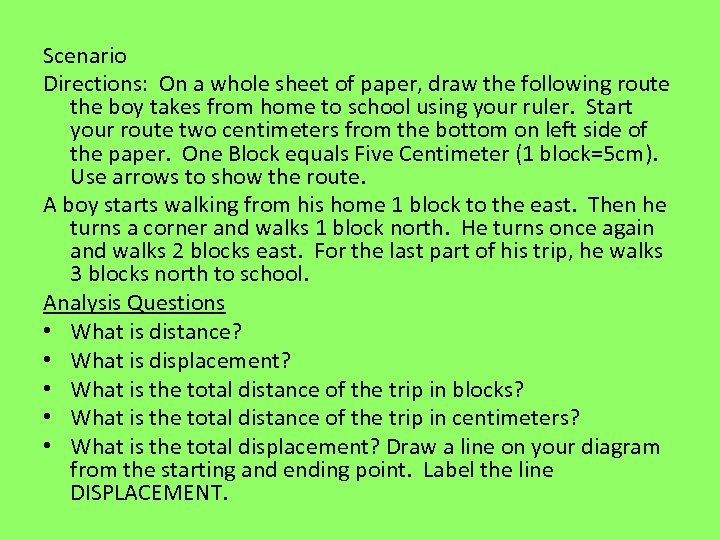Scenario Directions: On a whole sheet of paper, draw the following route the boy takes from home to school using your ruler. Start your route two centimeters from the bottom on left side of the paper. One Block equals Five Centimeter (1 block=5 cm). Use arrows to show the route. A boy starts walking from his home 1 block to the east. Then he turns a corner and walks 1 block north. He turns once again and walks 2 blocks east. For the last part of his trip, he walks 3 blocks north to school. Analysis Questions • What is distance? • What is displacement? • What is the total distance of the trip in blocks? • What is the total distance of the trip in centimeters? • What is the total displacement? Draw a line on your diagram from the starting and ending point. Label the line DISPLACEMENT.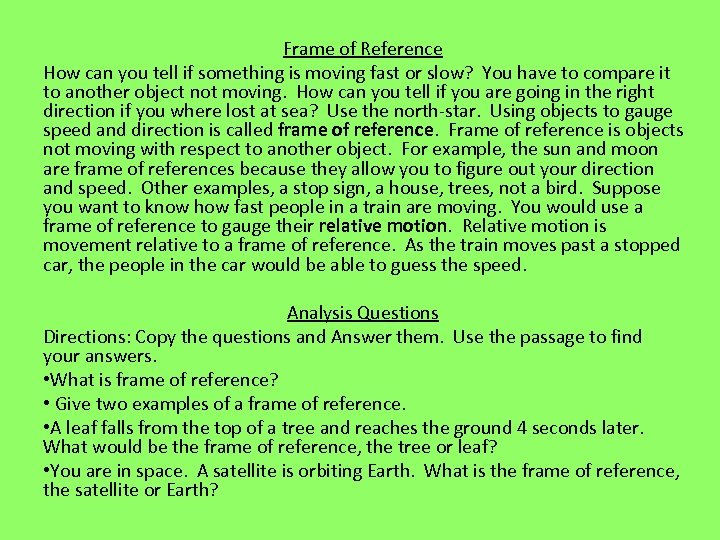Frame of Reference How can you tell if something is moving fast or slow? You have to compare it to another object not moving. How can you tell if you are going in the right direction if you where lost at sea? Use the north-star. Using objects to gauge speed and direction is called frame of reference. Frame of reference is objects not moving with respect to another object. For example, the sun and moon are frame of references because they allow you to figure out your direction and speed. Other examples, a stop sign, a house, trees, not a bird. Suppose you want to know how fast people in a train are moving. You would use a frame of reference to gauge their relative motion. Relative motion is movement relative to a frame of reference. As the train moves past a stopped car, the people in the car would be able to guess the speed. Analysis Questions Directions: Copy the questions and Answer them. Use the passage to find your answers. • What is frame of reference? • Give two examples of a frame of reference. • A leaf falls from the top of a tree and reaches the ground 4 seconds later. What would be the frame of reference, the tree or leaf? • You are in space. A satellite is orbiting Earth. What is the frame of reference, the satellite or Earth?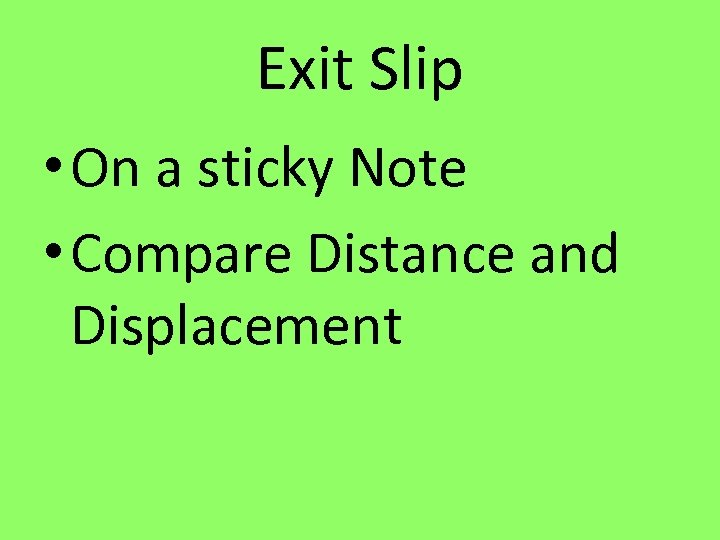Exit Slip • On a sticky Note • Compare Distance and Displacement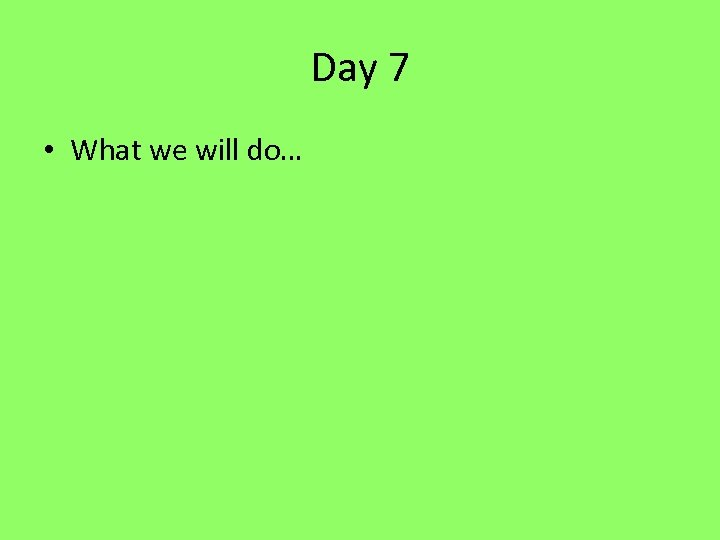Day 7 • What we will do…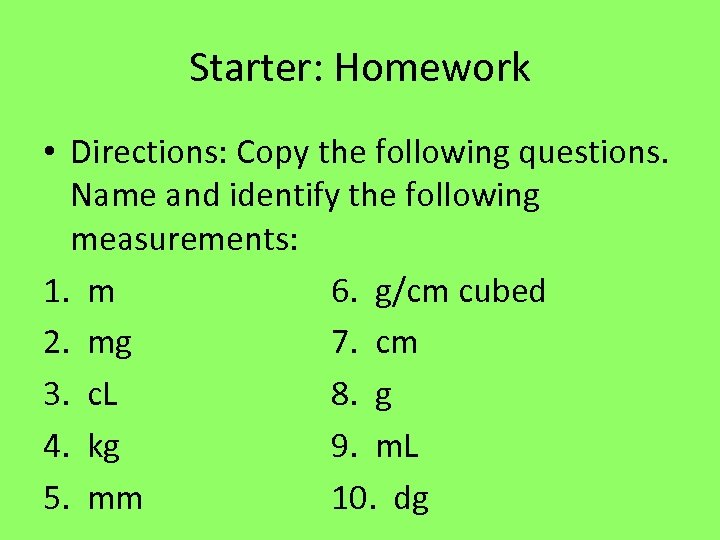Starter: Homework • Directions: Copy the following questions. Name and identify the following measurements: 1. m 6. g/cm cubed 2. mg 7. cm 3. c. L 8. g 4. kg 9. m. L 5. mm 10. dg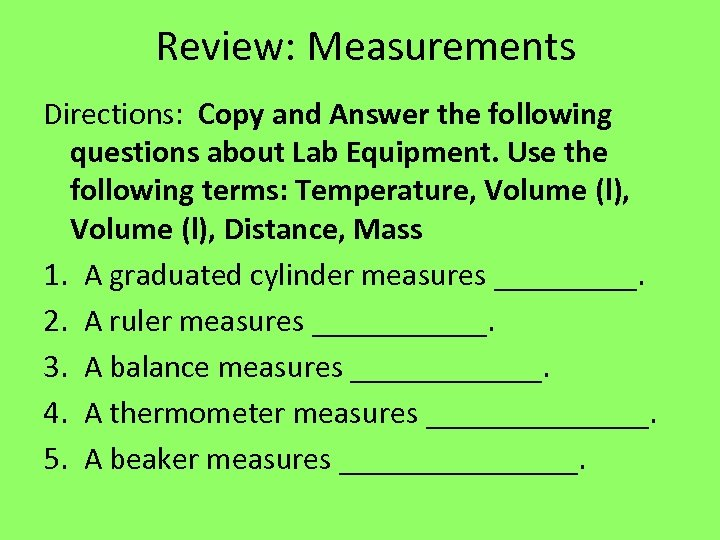Review: Measurements Directions: Copy and Answer the following questions about Lab Equipment. Use the following terms: Temperature, Volume (l), Distance, Mass 1. A graduated cylinder measures _____. 2. A ruler measures ______. 3. A balance measures ______. 4. A thermometer measures _______. 5. A beaker measures ________.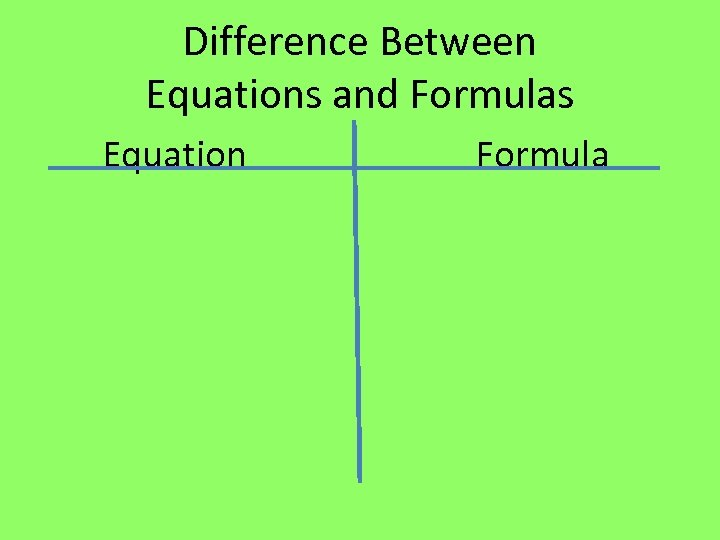Difference Between Equations and Formulas Equation Formula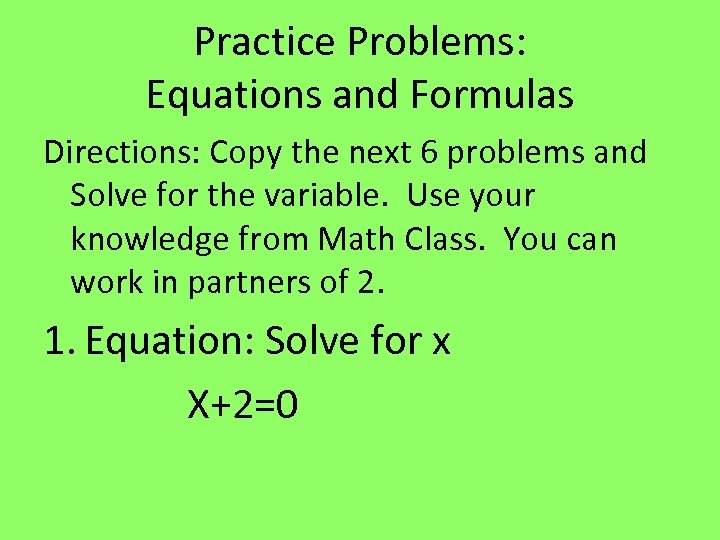Practice Problems: Equations and Formulas Directions: Copy the next 6 problems and Solve for the variable. Use your knowledge from Math Class. You can work in partners of 2. 1. Equation: Solve for x X+2=0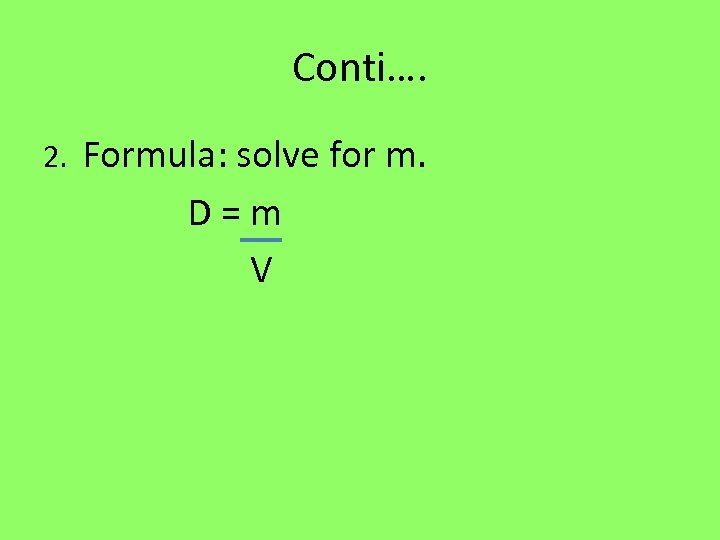Conti…. 2. Formula: solve for m. D = m V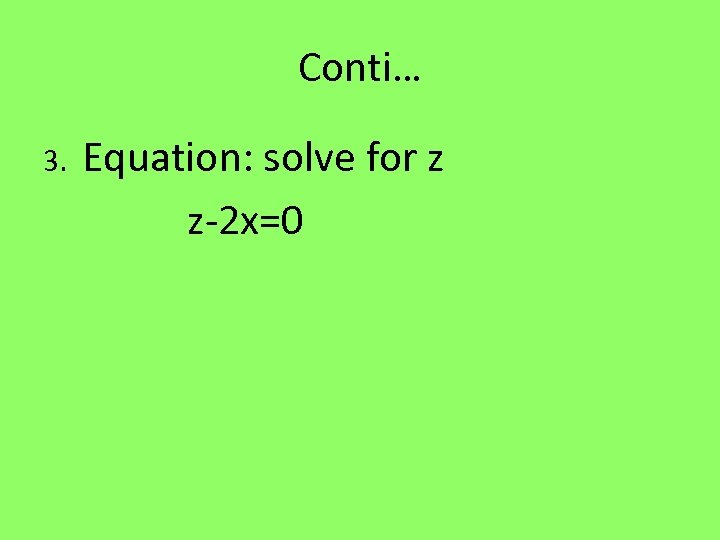Conti… 3. Equation: solve for z z-2 x=0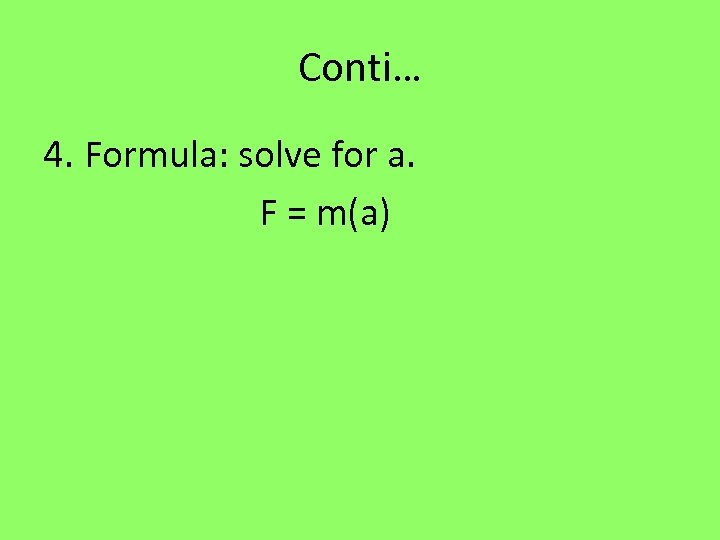Conti… 4. Formula: solve for a. F = m(a)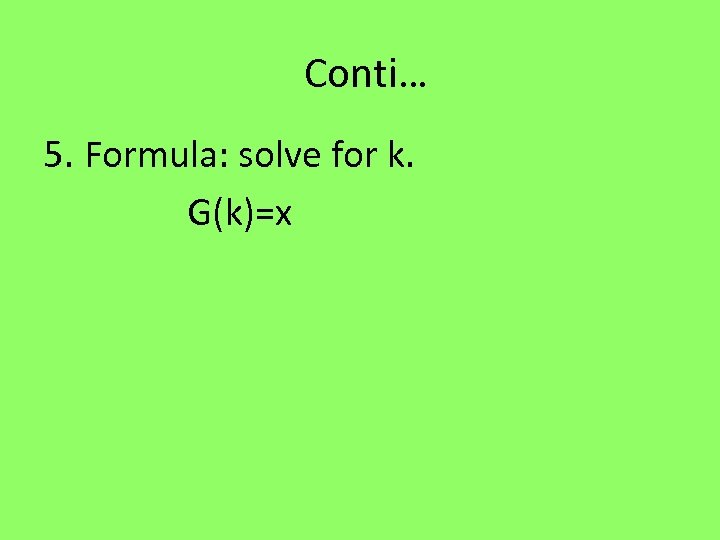Conti… 5. Formula: solve for k. G(k)=x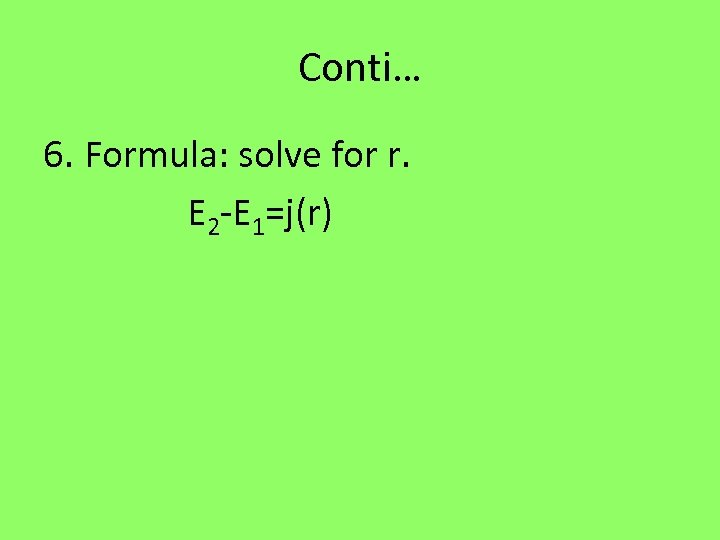Conti… 6. Formula: solve for r. E 2 -E 1=j(r)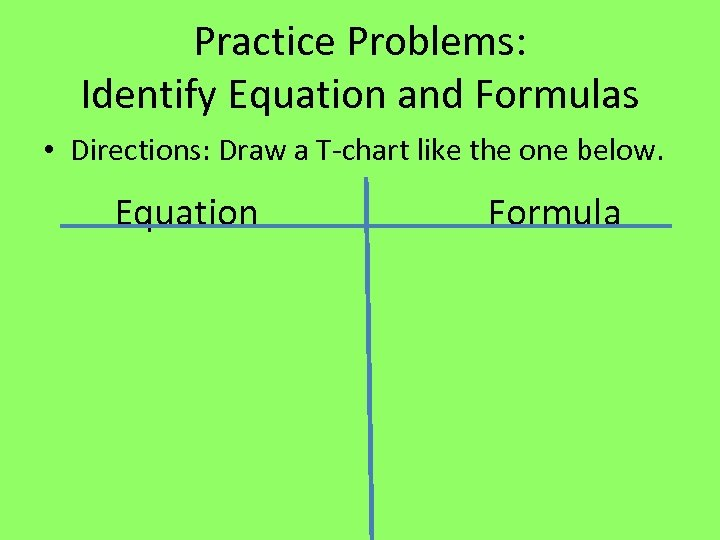Practice Problems: Identify Equation and Formulas • Directions: Draw a T-chart like the one below. Equation Formula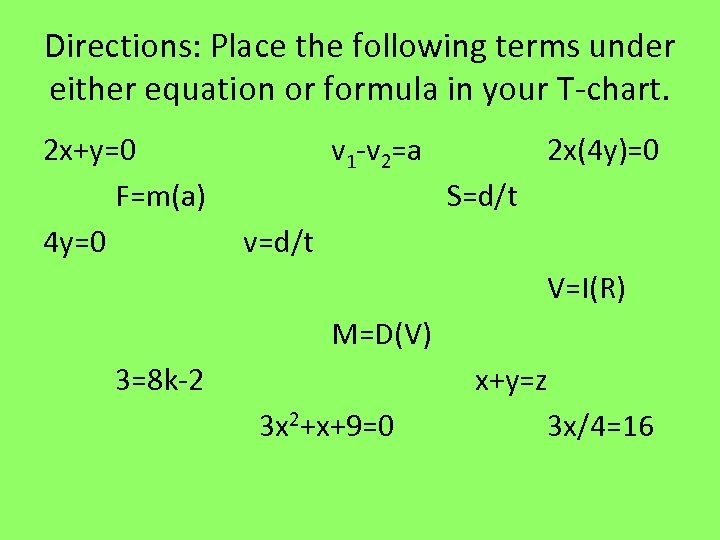Directions: Place the following terms under either equation or formula in your T-chart. 2 x+y=0 v 1 -v 2=a 2 x(4 y)=0 F=m(a) S=d/t 4 y=0 v=d/t V=I(R) M=D(V) 3=8 k-2 x+y=z 3 x 2+x+9=0 3 x/4=16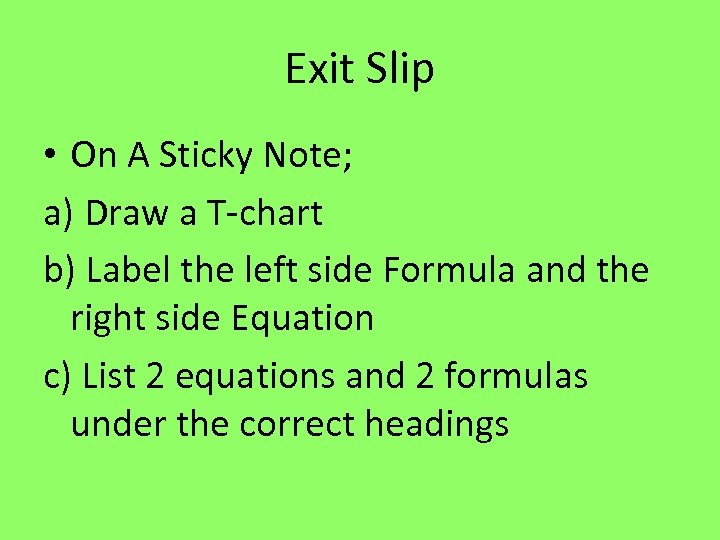Exit Slip • On A Sticky Note; a) Draw a T-chart b) Label the left side Formula and the right side Equation c) List 2 equations and 2 formulas under the correct headings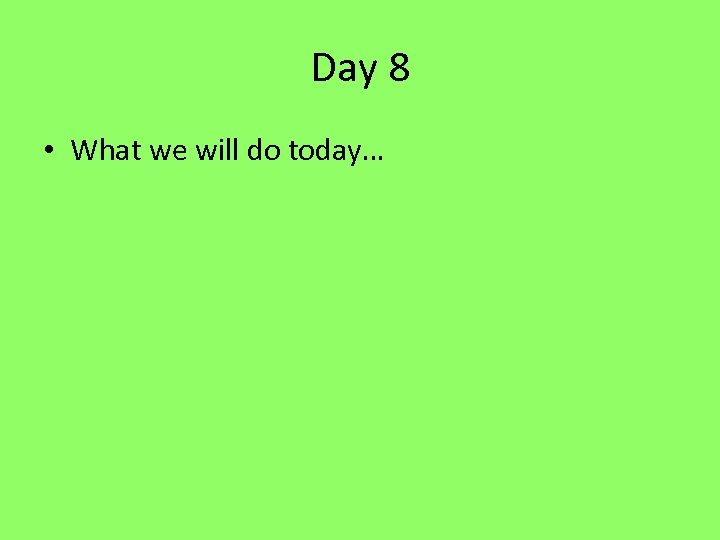Day 8 • What we will do today…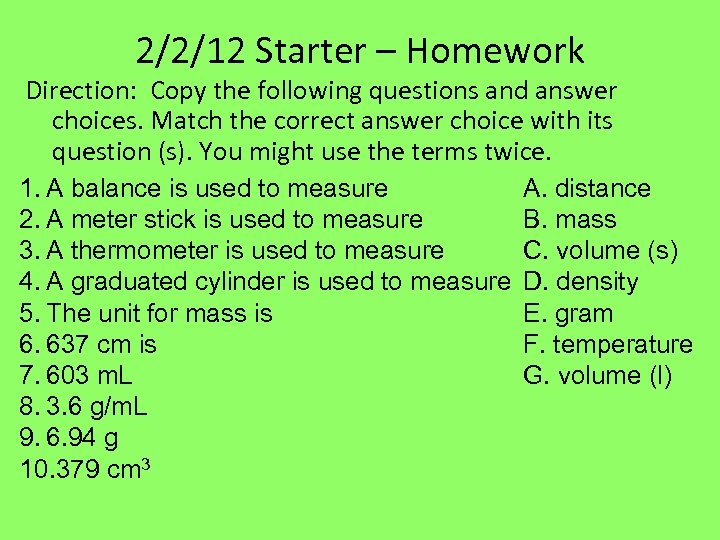2/2/12 Starter – Homework Direction: Copy the following questions and answer choices. Match the correct answer choice with its question (s). You might use the terms twice. 1. A balance is used to measure 2. A meter stick is used to measure 3. A thermometer is used to measure 4. A graduated cylinder is used to measure 5. The unit for mass is 6. 637 cm is 7. 603 m. L 8. 3. 6 g/m. L 9. 6. 94 g 10. 379 cm 3 A. distance B. mass C. volume (s) D. density E. gram F. temperature G. volume (l)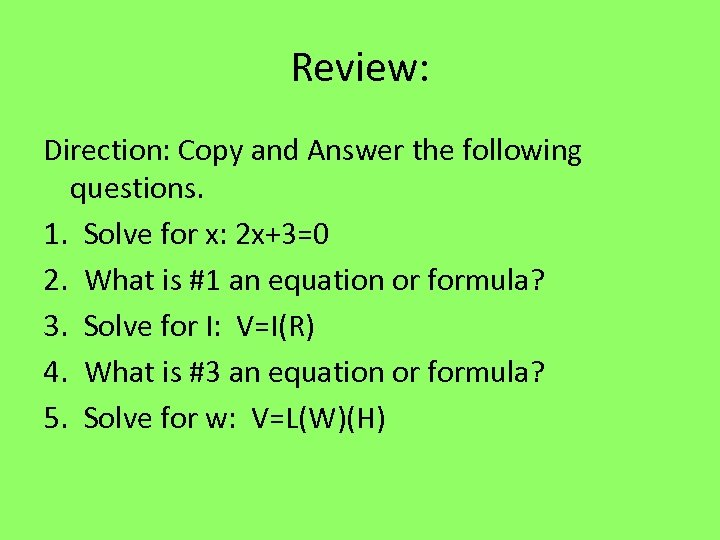Review: Direction: Copy and Answer the following questions. 1. Solve for x: 2 x+3=0 2. What is #1 an equation or formula? 3. Solve for I: V=I(R) 4. What is #3 an equation or formula? 5. Solve for w: V=L(W)(H)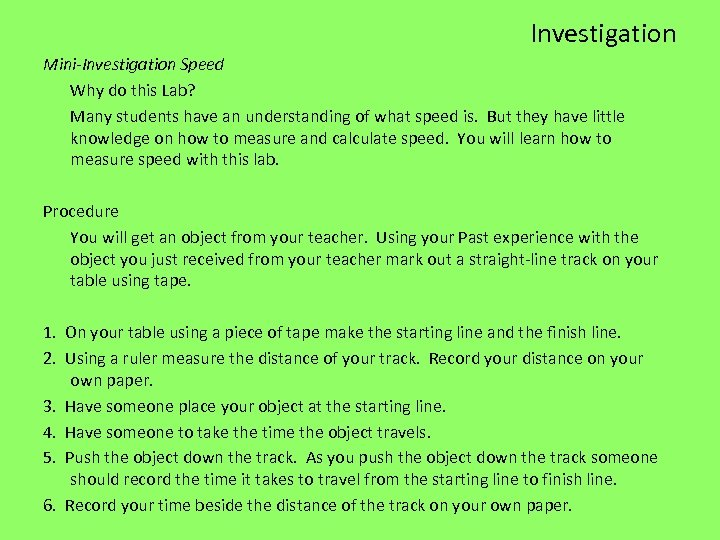Investigation Mini-Investigation Speed Why do this Lab? Many students have an understanding of what speed is. But they have little knowledge on how to measure and calculate speed. You will learn how to measure speed with this lab. Procedure You will get an object from your teacher. Using your Past experience with the object you just received from your teacher mark out a straight-line track on your table using tape. 1. On your table using a piece of tape make the starting line and the finish line. 2. Using a ruler measure the distance of your track. Record your distance on your own paper. 3. Have someone place your object at the starting line. 4. Have someone to take the time the object travels. 5. Push the object down the track. As you push the object down the track someone should record the time it takes to travel from the starting line to finish line. 6. Record your time beside the distance of the track on your own paper.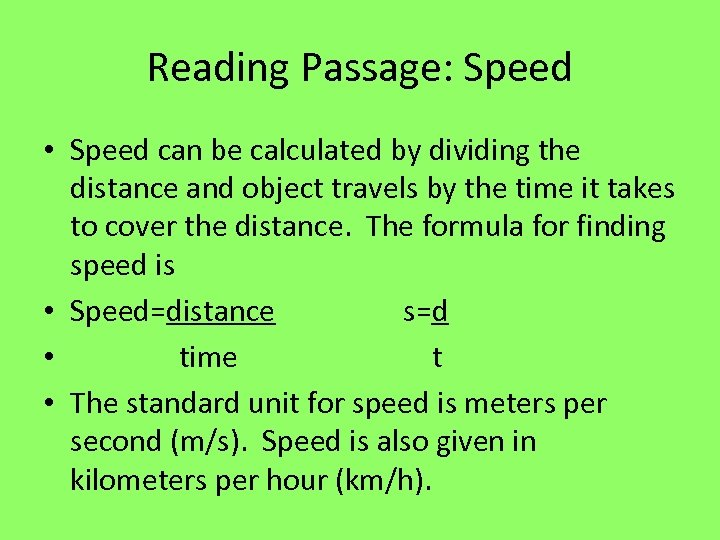Reading Passage: Speed • Speed can be calculated by dividing the distance and object travels by the time it takes to cover the distance. The formula for finding speed is • Speed=distance s=d • time t • The standard unit for speed is meters per second (m/s). Speed is also given in kilometers per hour (km/h).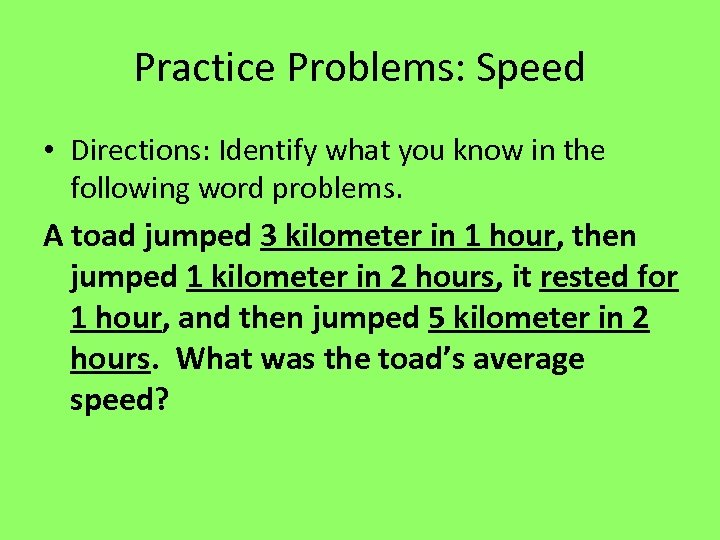Practice Problems: Speed • Directions: Identify what you know in the following word problems. A toad jumped 3 kilometer in 1 hour, then jumped 1 kilometer in 2 hours, it rested for 1 hour, and then jumped 5 kilometer in 2 hours. What was the toad’s average speed?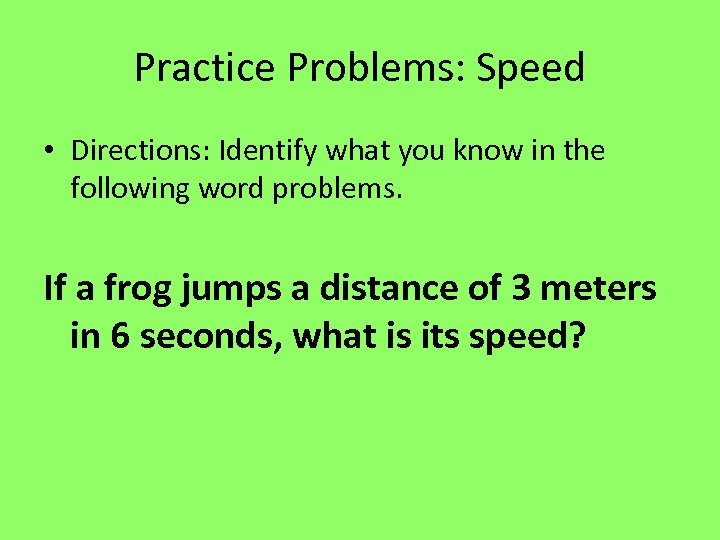Practice Problems: Speed • Directions: Identify what you know in the following word problems. If a frog jumps a distance of 3 meters in 6 seconds, what is its speed?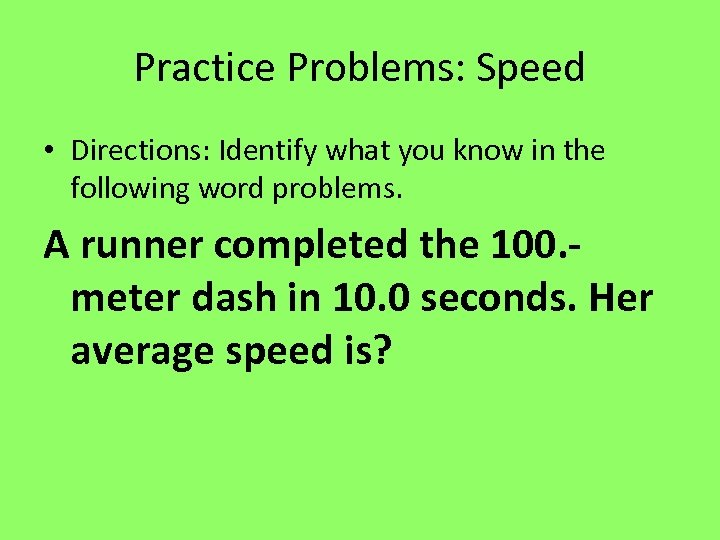Practice Problems: Speed • Directions: Identify what you know in the following word problems. A runner completed the 100. meter dash in 10. 0 seconds. Her average speed is?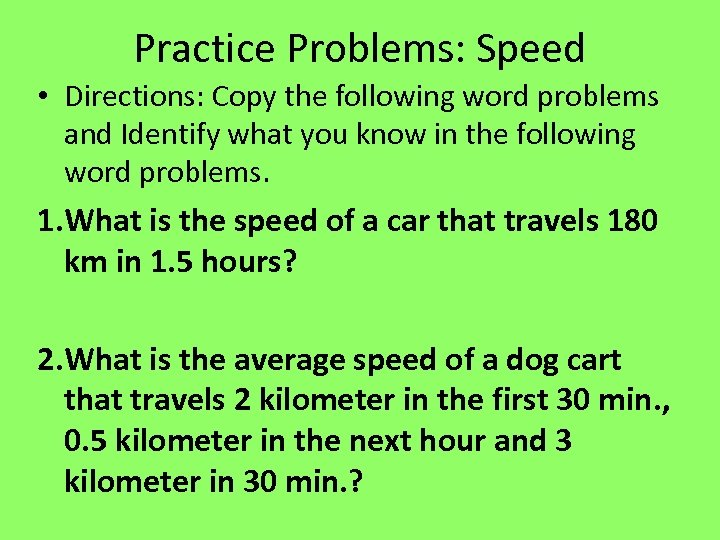Practice Problems: Speed • Directions: Copy the following word problems and Identify what you know in the following word problems. 1. What is the speed of a car that travels 180 km in 1. 5 hours? 2. What is the average speed of a dog cart that travels 2 kilometer in the first 30 min. , 0. 5 kilometer in the next hour and 3 kilometer in 30 min. ?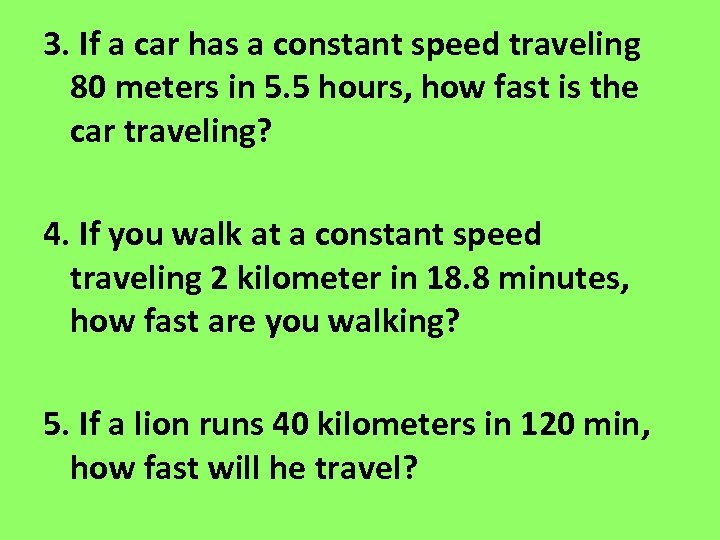3. If a car has a constant speed traveling 80 meters in 5. 5 hours, how fast is the car traveling? 4. If you walk at a constant speed traveling 2 kilometer in 18. 8 minutes, how fast are you walking? 5. If a lion runs 40 kilometers in 120 min, how fast will he travel?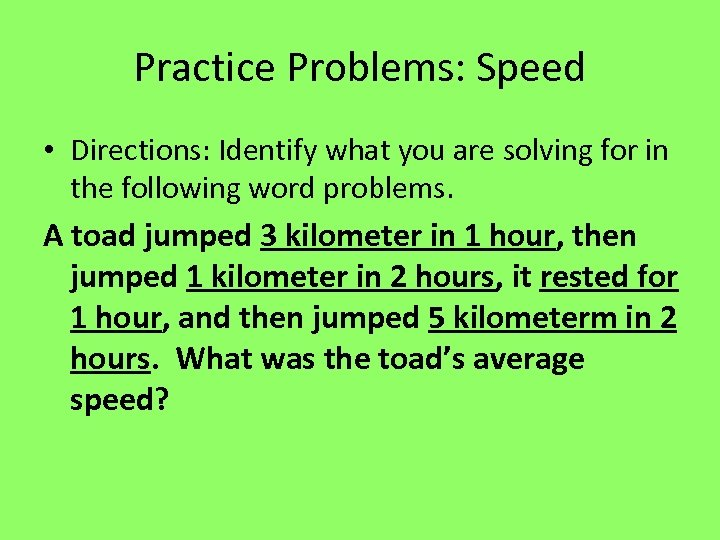Practice Problems: Speed • Directions: Identify what you are solving for in the following word problems. A toad jumped 3 kilometer in 1 hour, then jumped 1 kilometer in 2 hours, it rested for 1 hour, and then jumped 5 kilometerm in 2 hours. What was the toad’s average speed?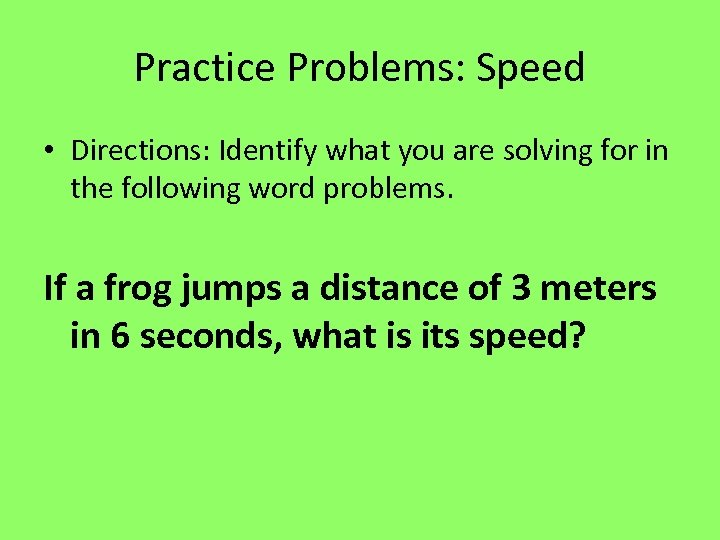Practice Problems: Speed • Directions: Identify what you are solving for in the following word problems. If a frog jumps a distance of 3 meters in 6 seconds, what is its speed?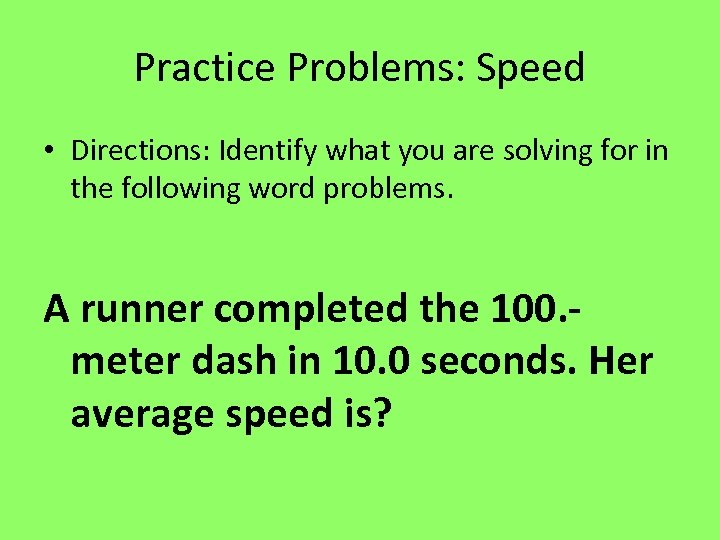Practice Problems: Speed • Directions: Identify what you are solving for in the following word problems. A runner completed the 100. meter dash in 10. 0 seconds. Her average speed is?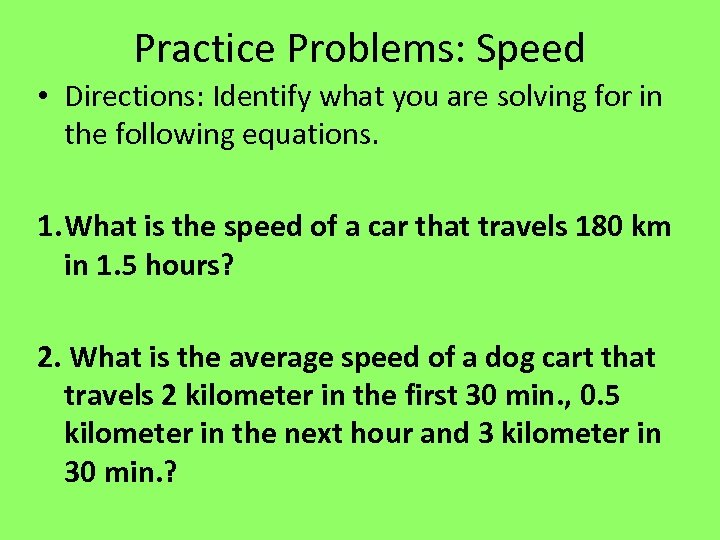Practice Problems: Speed • Directions: Identify what you are solving for in the following equations. 1. What is the speed of a car that travels 180 km in 1. 5 hours? 2. What is the average speed of a dog cart that travels 2 kilometer in the first 30 min. , 0. 5 kilometer in the next hour and 3 kilometer in 30 min. ?3. If a car has a constant speed traveling 80 meters in 5. 5 hours, how fast is the car traveling? 4. If you walk at a constant speed traveling 2 kilometer in 18. 8 minutes, how fast are you walking? 5. If a lion runs 40 kilometers in 120 min, how fast will he travel?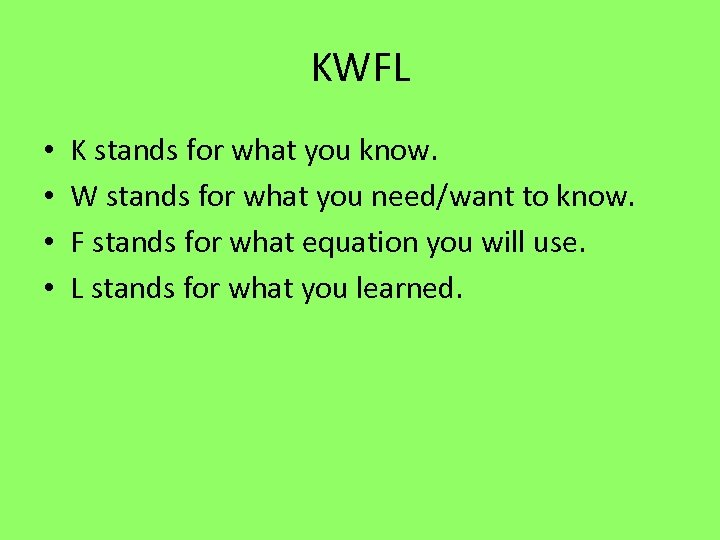KWFL • • K stands for what you know. W stands for what you need/want to know. F stands for what equation you will use. L stands for what you learned.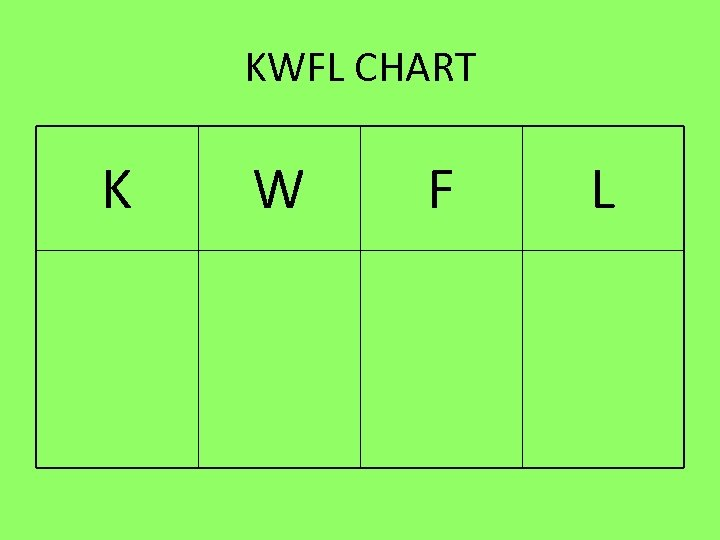KWFL CHART K W F L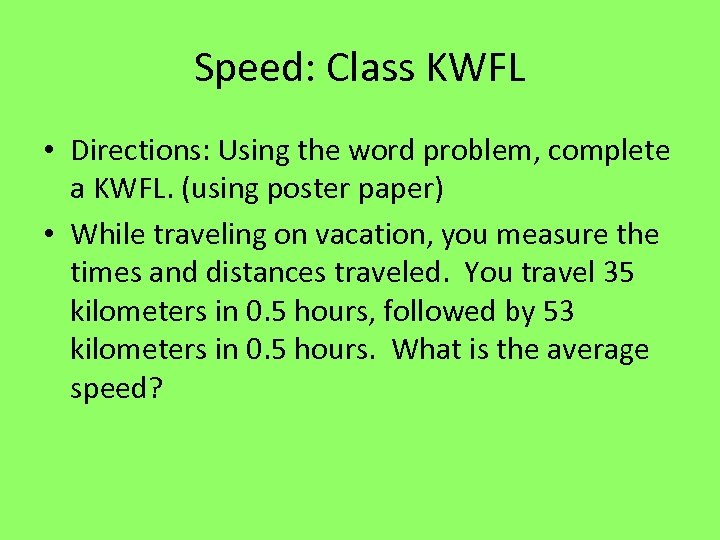Speed: Class KWFL • Directions: Using the word problem, complete a KWFL. (using poster paper) • While traveling on vacation, you measure the times and distances traveled. You travel 35 kilometers in 0. 5 hours, followed by 53 kilometers in 0. 5 hours. What is the average speed?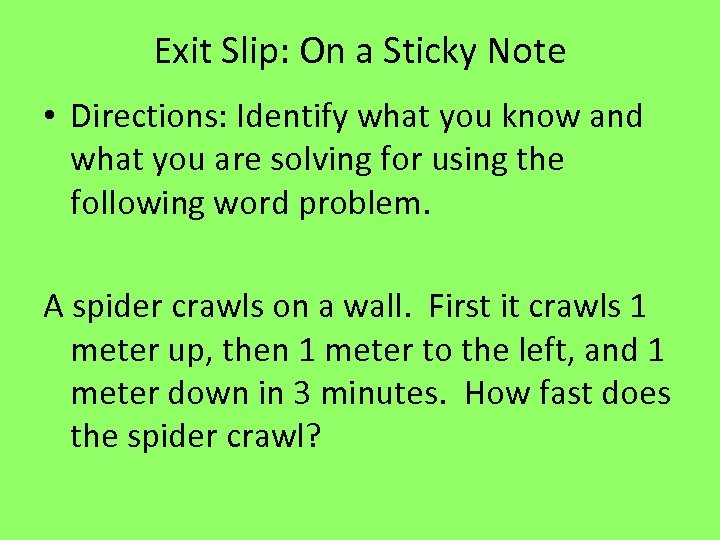Exit Slip: On a Sticky Note • Directions: Identify what you know and what you are solving for using the following word problem. A spider crawls on a wall. First it crawls 1 meter up, then 1 meter to the left, and 1 meter down in 3 minutes. How fast does the spider crawl?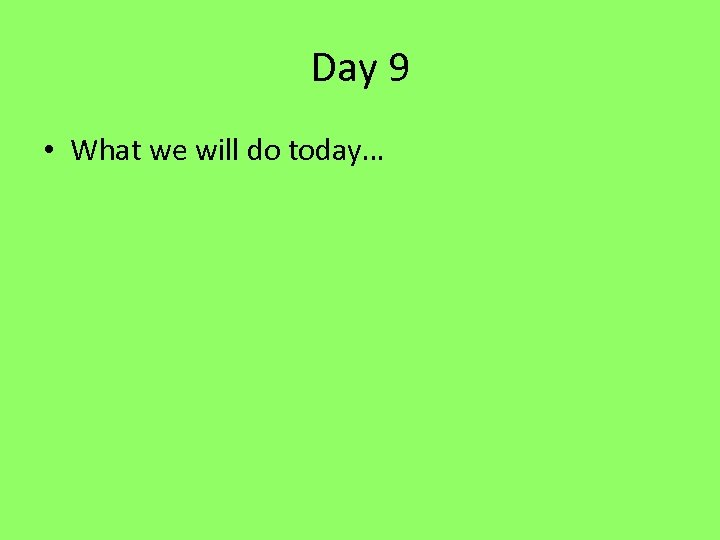Day 9 • What we will do today…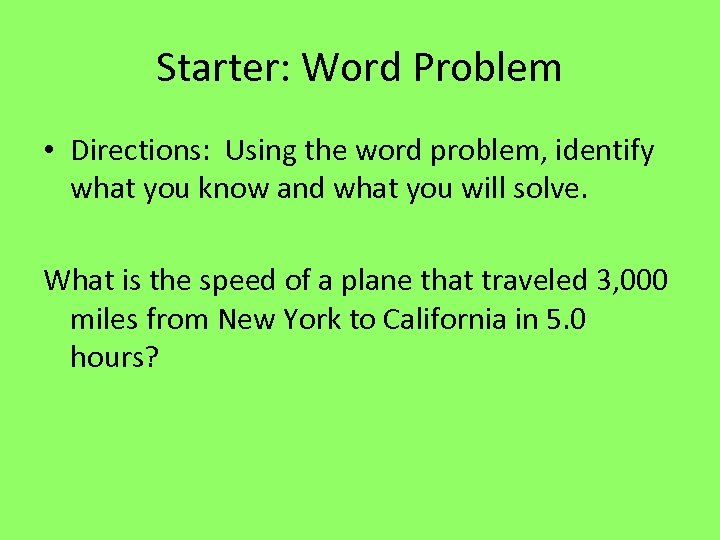Starter: Word Problem • Directions: Using the word problem, identify what you know and what you will solve. What is the speed of a plane that traveled 3, 000 miles from New York to California in 5. 0 hours?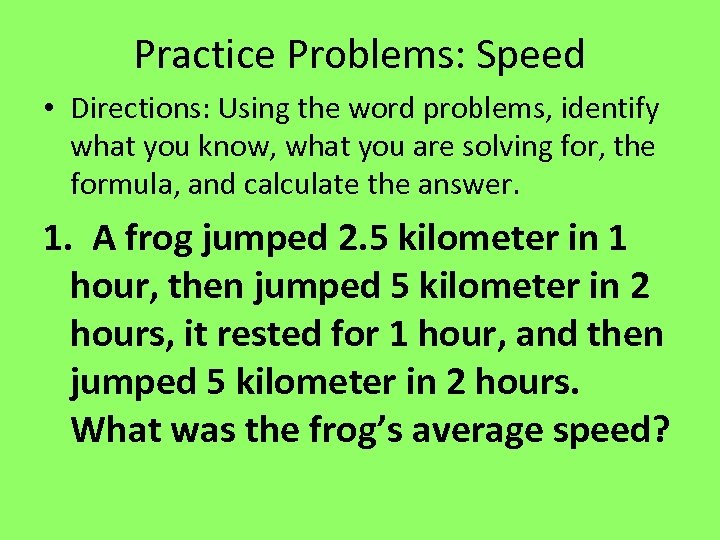Practice Problems: Speed • Directions: Using the word problems, identify what you know, what you are solving for, the formula, and calculate the answer. 1. A frog jumped 2. 5 kilometer in 1 hour, then jumped 5 kilometer in 2 hours, it rested for 1 hour, and then jumped 5 kilometer in 2 hours. What was the frog’s average speed?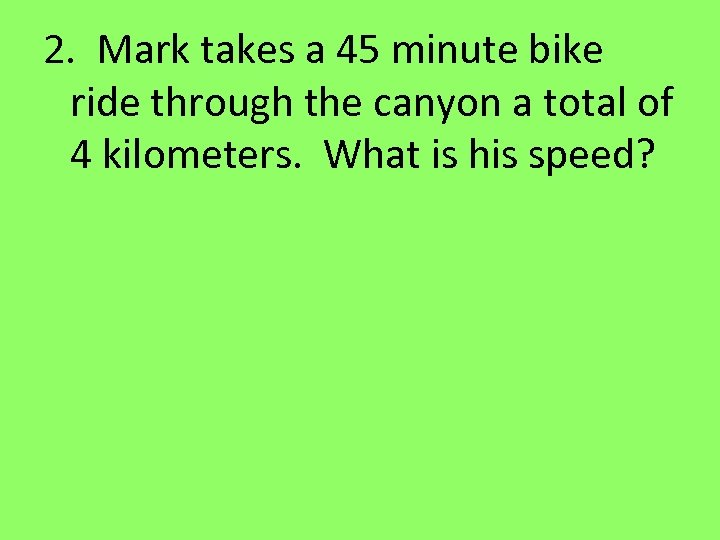2. Mark takes a 45 minute bike ride through the canyon a total of 4 kilometers. What is his speed?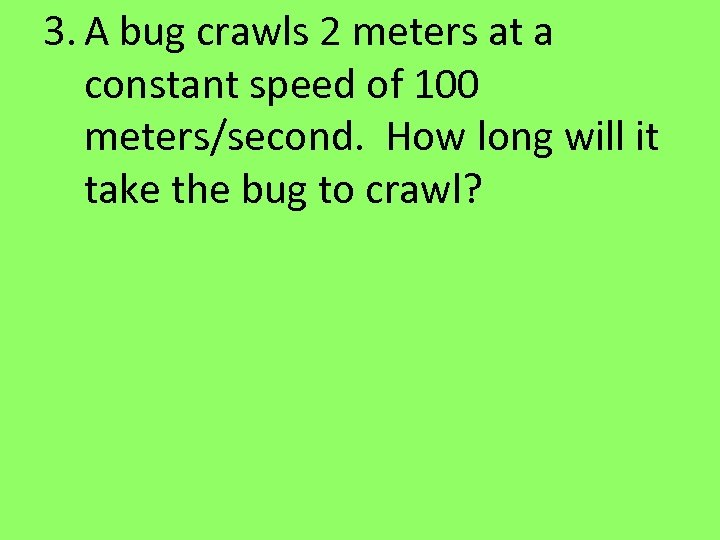3. A bug crawls 2 meters at a constant speed of 100 meters/second. How long will it take the bug to crawl?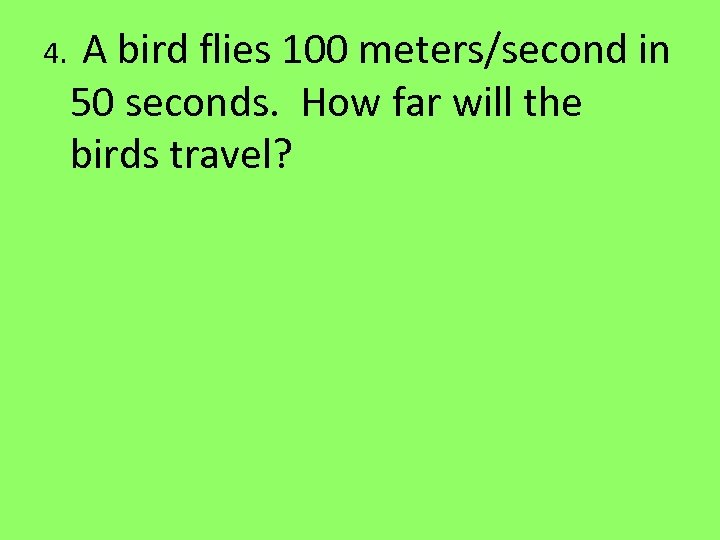4. A bird flies 100 meters/second in 50 seconds. How far will the birds travel?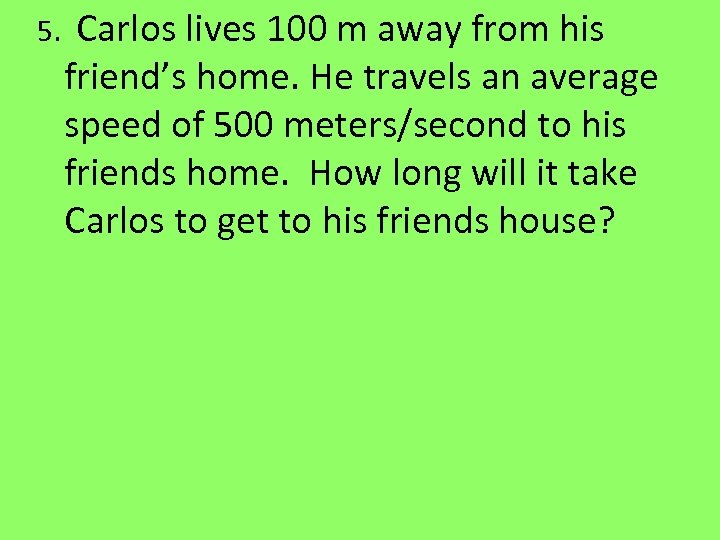5. Carlos lives 100 m away from his friend’s home. He travels an average speed of 500 meters/second to his friends home. How long will it take Carlos to get to his friends house?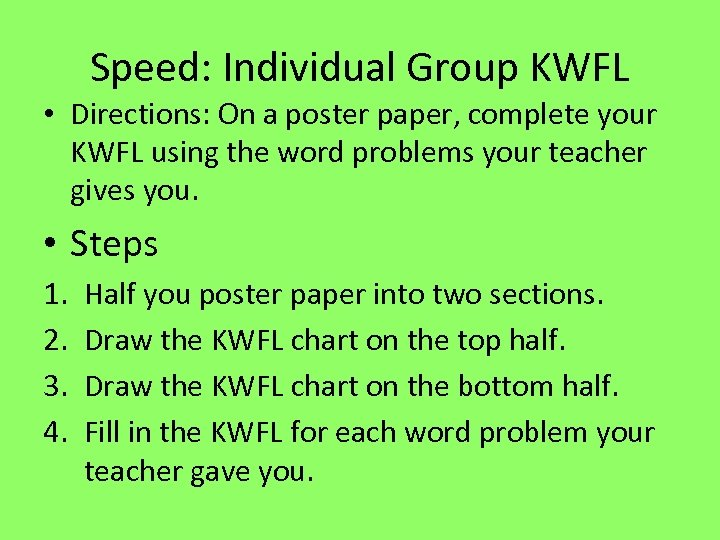Speed: Individual Group KWFL • Directions: On a poster paper, complete your KWFL using the word problems your teacher gives you. • Steps 1. 2. 3. 4. Half you poster paper into two sections. Draw the KWFL chart on the top half. Draw the KWFL chart on the bottom half. Fill in the KWFL for each word problem your teacher gave you.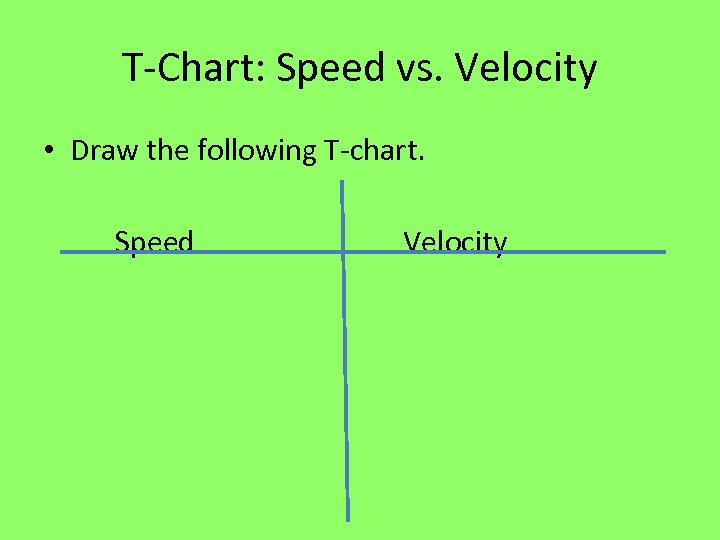T-Chart: Speed vs. Velocity • Draw the following T-chart. Speed Velocity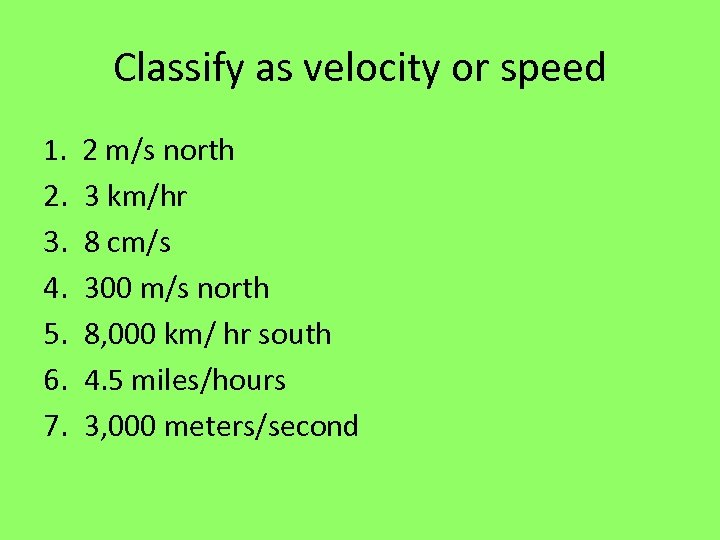Classify as velocity or speed 1. 2 m/s north 2. 3 km/hr 3. 8 cm/s 4. 300 m/s north 5. 8, 000 km/ hr south 6. 4. 5 miles/hours 7. 3, 000 meters/second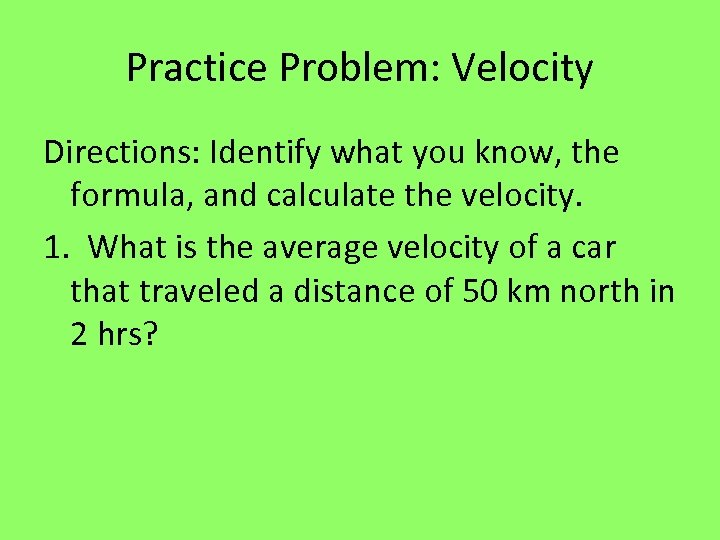Practice Problem: Velocity Directions: Identify what you know, the formula, and calculate the velocity. 1. What is the average velocity of a car that traveled a distance of 50 km north in 2 hrs?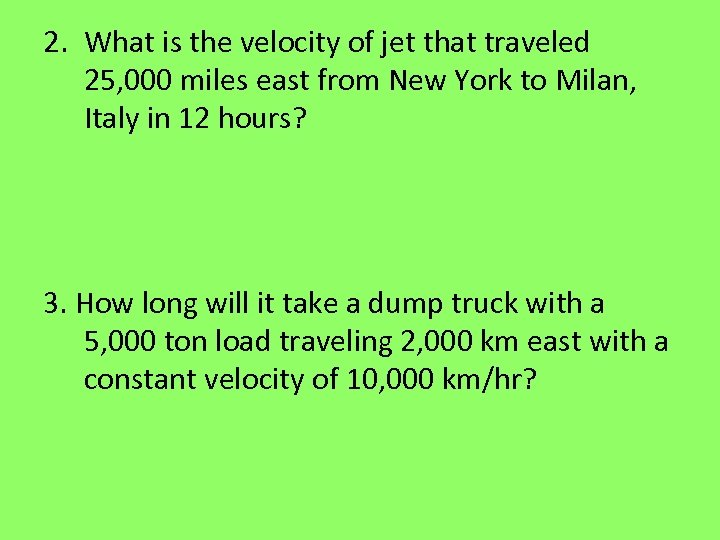2. What is the velocity of jet that traveled 25, 000 miles east from New York to Milan, Italy in 12 hours? 3. How long will it take a dump truck with a 5, 000 ton load traveling 2, 000 km east with a constant velocity of 10, 000 km/hr?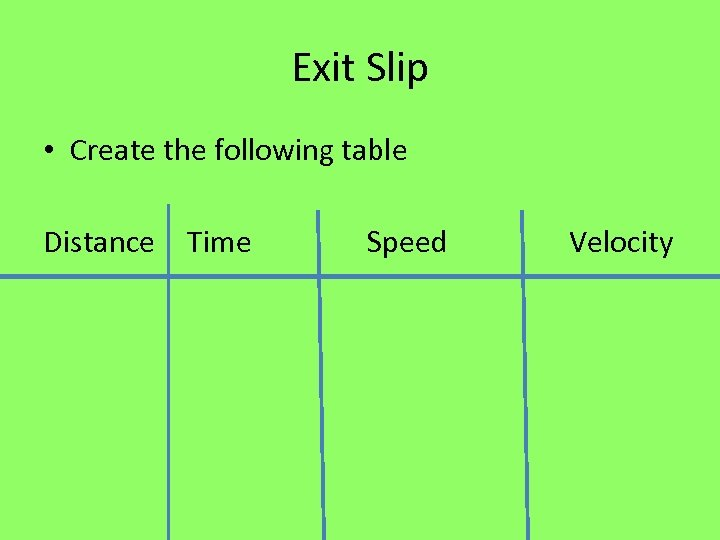Exit Slip • Create the following table Distance Time Speed Velocity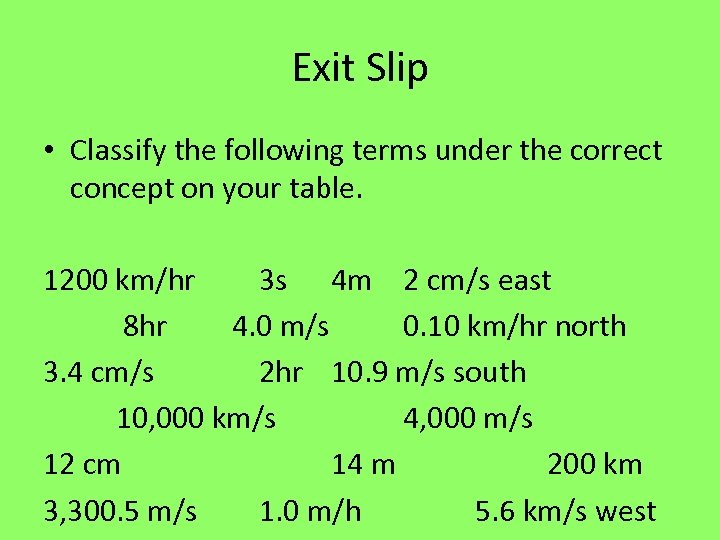Exit Slip • Classify the following terms under the correct concept on your table. 1200 km/hr 3 s 4 m 2 cm/s east 8 hr 4. 0 m/s 0. 10 km/hr north 3. 4 cm/s 2 hr 10. 9 m/s south 10, 000 km/s 4, 000 m/s 12 cm 14 m 200 km 3, 300. 5 m/s 1. 0 m/h 5. 6 km/s west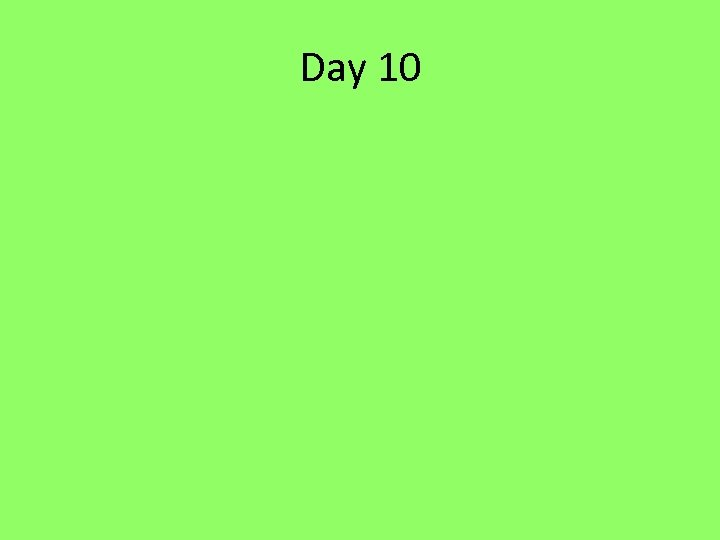Day 10